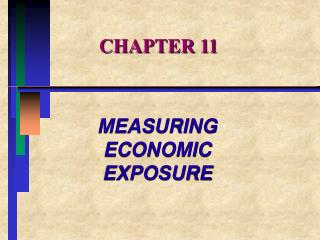DownloadDownload PresentationCHAPTER 11

# CHAPTER 11

Télécharger la présentation## CHAPTER 11

- - - - - - - - - - - - - - - - - - - - - - - - - - - E N D - - - - - - - - - - - - - - - - - - - - - - - - - - -
##### Presentation Transcript

1. CHAPTER 11 MEASURING ECONOMIC EXPOSURE

2. CHAPTER OVERVIEW • I. Foreign Exchange Risk and Economic Exposure • II. The Economic Consequences of Exchange Rate Changes • III . Identifying Economic Exposure • IV. Calculating Economic Exposure • V. An Operational Measure of Exchange Rate Risk

3. Part I. Foreign Exchange Risk and Economic Exposure • I. FOREIGN EXCHANGE RISK • A. Economic exposure • focuses on the impact of currency fluctuations on firm’s value. • 1 . Expectations about the fluctuation must be incorporated in all basic • decisions of the firm.

4. FOREIGN EXCHANGE RISK AND ECONOMIC EXPOSURE • 2. Definitions: • a. Accounting exposure • impact on firm’s balance sheet • b. Economic exposure • 1.) Transaction • 2.) Operating

5. FOREIGN EXCHANGE RISK AND ECONOMIC EXPOSURE • THE REAL EXCHANGE RATE

6. FOREIGN EXCHANGE RISK AND ECONOMIC EXPOSURE • B. Real Exchange Rates and Risk • 1. Nominative v. real exchange rates • real rate has been adjusted for • price changes. • 2. Hobson’s Choice: • when faced with a change in real value, • do you keep price constant (changing sales) or change prices (change profits)

7. FOREIGN EXCHANGE RISK AND ECONOMIC EXPOSURE • 3. SUMMARY • a. the economic impact of a currency change depends on the offset by the difference in inflation rates or the real exchange rate. • b. It is the relative price changes that ultimately determine a firm’s long-run exposure.

8. PART II. THE ECONOMIC CONSEQUENCES OF EXCHANGE RATE CHANGES • II. ECONOMIC CONSEQUENCES • A. Transaction exposure • 1. On-balance sheet • 2. Off-balance sheet

9. THE ECONOMIC CONSEQUENCES OF EXCHANGE RATE CHANGES • II. ECONOMIC CONSEQUENCES (con’t) • B. Operating Exposure : real rate change • 1. Pricing flexibility is key. • 2. Product differentiation • 3. Substitution of inputs

10. THE ECONOMIC CONSEQUENCES OF EXCHANGE RATE CHANGES • II. SUMMARY • The sector of the economy in which the firm operates; • the sources of the firm’s inputs; • and • fluctuations in the real exchange rate • delineate the firm’s true economic • exposure.

11. PART III. IDENTIFYING ECONOMIC EXPOSURE • III. CASE STUDIES OF ECONOMIC EXPOSURE • A. ASPEN SKIING COMPANY • 1. Firm’s exchange rate risk affected • its sales revenues.

12. PART III. IDENTIFYING ECONOMIC EXPOSURE • A. ASPEN SKIING COMPANY (con’t) • 2. Although there was no translation • risk, the global market with its exchange rate risk and its competitors impacted market demand

13. PART III. IDENTIFYING ECONOMIC EXPOSURE • B. PETROLEOS MEXICANOS (PEMEX) • 1. The firm’s exchange rate risk • affected cost but not revenues. • 2. Economic impact • a. Revenues: none • b. Costs: decreased • c. Net effect: increased US\$ flows

14. IDENTIFYING ECONOMIC EXPOSURE • C. TOYOTA MOTOR COMPANY • 1. Exchange rate risk affected BOTH • revenues and costs. • 2. Flow back effect: • previously exported goods return • with increased domestic competition. • 3. Lower profit margins domestically

15. PART IV. CALCULATING ECONOMIC EXPOSURE • IV. A quantitative assessment of economic • exposure depends on underlying assumptions concerning: • A. future cash flows; • B. sensitivity to exchange rate • changes.

16. PART V. AN OPERATIONAL MEASURE OF EXCHANGE RISK • V. NEED FOR A WORKABLE APPROACH • A. Regression Analysis • 1. Variables • a. Independent: • changes in parent’s cash flows • b. Dependent: • Average nominal exchange rate change.

17. AN OPERATIONAL MEASURE OF EXCHANGE RISK • B. REGRESSION EQUATION • -approach based on the operational definition of the exchange risk faced by a parent or one of its affiliates: • -a company faces exchange risk to the extent that variations in the dollar value of the unit’s cash flows are correlated with variations in the nominal exchange rate

18. AN OPERATIONAL MEASURE OF EXCHANGE RISK • where CFt = CFt - CFt-1 is the dollar value of total affiliate(parent) cash flows in period t • EXCHt = EXCHt - EXCHt-1 equals the average nominal exchange rate during period t • u = a random error term

19. AN OPERATIONAL MEASURE OF EXCHANGE RISK • 1. Output measures: • a. Beta coefficient (b) • measures the association of changes in cash flows to exchange rate changes.

20. AN OPERATIONAL MEASURE OF EXCHANGE RISK • b. the higher the percentage change of cash flow to changes in exchange rates, the greater the economic exposure (higher beta values).

21. AN OPERATIONAL MEASURE OF EXCHANGE RISK • VI. SUMMARY: • A. The focus of the accounting profession on the balance sheet impact of currency changes has led to ignoring the important impact on future cash flows.

22. AN OPERATIONAL MEASURE OF EXCHANGE RISK • B. For firms incurring costs and selling products in foreign countries, the net effect of currency changes may be less important in the long run.

23. AN OPERATIONAL MEASURE OF EXCHANGE RISK • C. To measure exposure properly, you must focus on inflation-adjusted or real exchange rates instead of nominal or actual exchange rates.

24. AN OPERATIONAL MEASURE OF EXCHANGE RISK • D. It is difficult in practice to determine what the actual economic impact of a currency change will be.